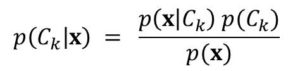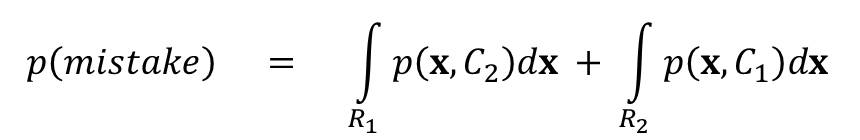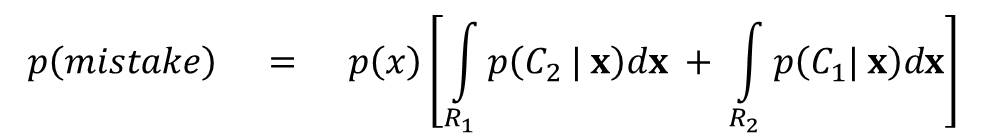# Machine Learning 101 – Minimizing Misclassification Rate in Bayes’ Classifier

In Lecture 4, we learnt about the Bayes’ classifier. Here  we would see how to minimize misclassfication rate in Bayes classifier. Again, we would review the cancer diagnosis example.

Review of Cancer Diagnosis Example

In this example, the doctors need to determine if the patient has cancer or now. To make this decision, they take an X-ray image of the patient. The output of this image is represented a a vector x which represents a set of pixels from the X-ray image.

So this is a typical classification problem.  They need to classify the patient into one of two classes:

• C1 – cancer present
• C2 – cancer absent

If we let k = 1, 2, then we can represent the classes as Ck.

So, given the patient’s X-ray image x, what is the probability of Ck (that is cancer is present or absent)?

We can represent this as:

p(Ck | x)

Do you remember that this is conditional probability?

And by Bayes’ Theorem, this is gotten using the formula:When Misclassification occurs

Misclassification occurs if cancer is present but the doctors decide that it is not.

Representing this with notations, we say that:

• misclassification occurs if the patient belongs to class C2, but the doctors assign him to C1 (false positive)
• Or the patient belongs to C1 but the doctors assign him to C2 (false negative)

Misclassification Rate

This is number of misclassifications divided by total number evaluated

#### Minimizing Misclassification Rate

First, we need to partition the input space into regions. (input space is all possible values x can take).

These regions are called decision regions. Let’s represent them using Rk. This is because, the number of decision regions equals number of classes. So one region for each class.

Now, all points in Rk are assigned to Ck.

The boundary between the decision regions are known as decision surfaces or decision boundaries.

So we need to a rule that would take each input x, and assign it to a region.

From our example, a mistake can occur in two possible ways:

• x belongs to R1 but assigned to C2
• x belongs to R2 bu assigned to C2

The probability of a mistake occurring is now, the sum of this two probabilities (remember the sum rule). This is given as

p(mistake) = p(x ∈ R1, C2) + p(x ∈ R2, C1)

This can be broken down by taking these probabilities over the regions R1, and R2. Therefor we have:Clearly, to  minimize p(mistake), we need to try to assign x to whichever class has the smaller value of the integrand.

Therefore, if p(x, C1) < p(x, C2) for a given x, then we should assign x to C1.

Let’s evaluate p(mistake) further using the product rule.

We know from the product rule that:

p(x, C2) = p(C2 | x)p(x)

also

p(x, C1) = p(C1 | x ) p(x)

If we use this in the formula for p(mistake), we would then have:You can now see that the terms in the integral is either p(C2 | x) dx or

p(C1 | x) dx

These conditional probabilities can work if we already know the posterior probabilities of C1 and C2 after obtaining x.

Therefore, the probability  of misclassification p(mistake) is minimized if each value of x is assigned to the class with the largest posterior probability.

Probability of Correct Classification

Similarly, we can apply the same method to obtain p(correct).

I recommend this as an exercise for you.#### kindsonthegenius

Kindson Munonye is currently completing his doctoral program in Software Engineering in Budapest University of Technology and Economics

View all posts by kindsonthegenius →